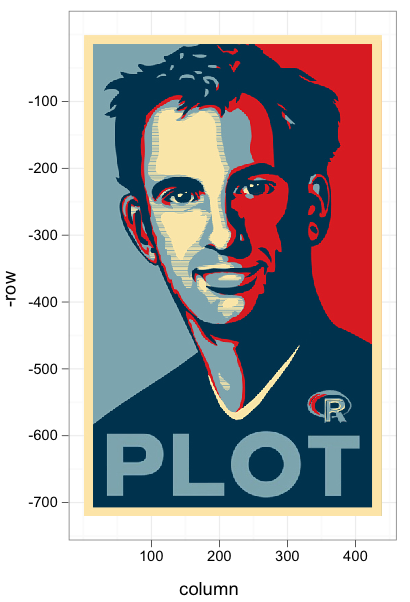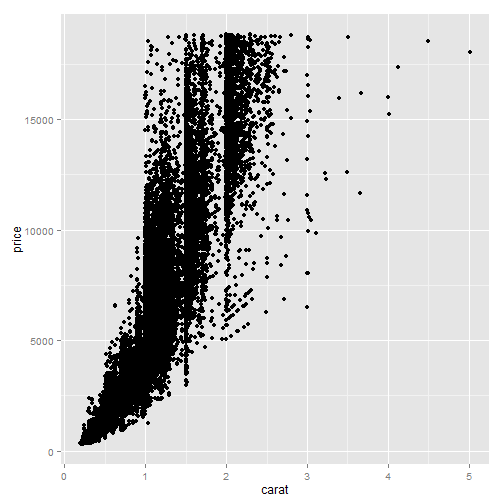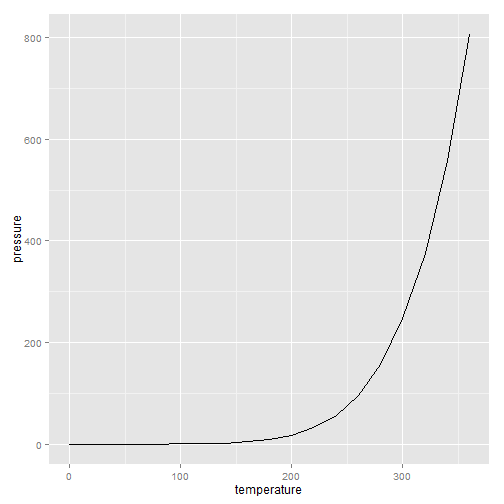# InfoViz with R

12th March 2015

### Introduction

This presentation is about visualizing data with R

• We are going to use R package ggplot2
• We will talk about some principles of Information Visualization.

-Adapted from Dawn Koffman Office of Population Research Princeton University

### R Package ggplot2• Based on The Grammar of Graphics by Leland Wilkinson, 2005

### qplot()

ggplot2 provides two ways to produce plot objects:

• qplot() #quick plot Not covered in this presentation
• borrows some concepts from graphics of grammer but limited in capability
• very easy to produce basic graphs

• ggplot() grammar of graphics

• has a steeper learning curve but extremely powerful

### Grammar Defines Components of Graphics

data: in ggplot2, data must be stored as an R data frame

coordinate system: decribes 2-D space that data is projected onto

geoms: describe type of geometric objects that represent data

aesthetics: describe visual characteristics that represent data

scales: for each aesthetic, describe how visual characteristic is converted to display values

stats: describe statistical transformations that typically summarize data

facets: describe how data is split into subsets and displayed as multiple small graphs

### First Dive into ggplot2

``````#install.packages("ggplot2")
library(ggplot2)
ggplot(diamonds, aes(x=carat, y=price)) + geom_point()
``````### Creating a Line Graph

``````ggplot(pressure, aes(x=temperature, y=pressure)) + geom_line()
``````### Diamond Smoothing

``````ggplot(diamonds,aes(x=carat, y=price))+ geom_point()+ stat_smooth()
``````